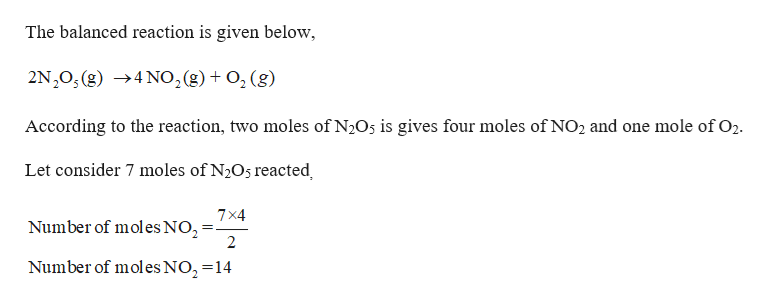# Calculate how many moles of NO2 form when each quantity of reactant completely reacts via the following reaction: 2N2O5(g)→4NO2(g)+ O2(g)16.0 g N2O5and 3.51 kg N2O5

Question
16 views

Calculate how many moles of NO2 form when each quantity of reactant completely reacts via the following reaction: 2N2O5(g)→4NO2(g)+ O2(g)

16.0 g N2O5

and

3.51 kg N2O5

check_circle

Step 1

Given,

N2O5 = 16.0 g,

N2O5 = 16.0 g,

Molar mass of N2O5 = 108.01 g...help_outlineImage TranscriptioncloseThe balanced reaction is given below 2N20, (g) 4 N02(g) 02 (g) According to the reaction, two moles of N2O5 is gives four moles of NO2 and one mole of O2. Let consider 7 moles of N2O5 reacted 7x4 Number of mol es NO Number of mol es NO, =14 fullscreen

### Want to see the full answer?

See Solution

#### Want to see this answer and more?

Solutions are written by subject experts who are available 24/7. Questions are typically answered within 1 hour.*

See Solution
*Response times may vary by subject and question.
Tagged in

### Chemistry